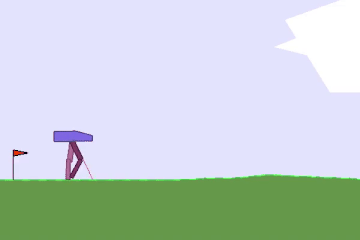• +91-9872993883
• +91-8283824812
• info@ris-ai.com

# BipedalWalker-v2 ¶

## What is BipedalWalker? ¶

Reward is given for moving forward, total 300+ points up to the far end. If the robot falls, it gets -100. Applying motor torque costs a small amount of points, more optimal agent will get better score. State consists of hull angle speed, angular velocity, horizontal speed, vertical speed, position of joints and joints angular speed, legs contact with ground, and 10 lidar rangefinder measurements. There's no coordinates in the state vector.

The problem is posed as a finite-horizon, non-deterministic Markov decision process (MDP), and is as interesting as it is difficult. The high dimensionality and continuous ranges of inputs (space) and outputs (actions) poses especially challenging examples of the lemmas of delayed reward, credit assignment, and exploration vs. exploitation.

Moreover, while the MDP might guarantee convergence to a deterministic optimal policy in the limit, the dimensionality and continuous range poses the challenge that it cannot be enumerated in finite space complexity.### Here are the steps for the movement of the BipedalWalker: ¶

In [ ]:
import numpy as np
import gym

from keras.models import Sequential, Model
from keras.layers import Dense, Activation, Flatten, Input, Concatenate

from rl.agents import DDPGAgent
from rl.memory import SequentialMemory
from rl.random import OrnsteinUhlenbeckProcess
ENV_NAME = 'BipedalWalker-v2'
# Get the environment and extract the number of actions.
env = gym.make(ENV_NAME)

In [ ]:
## play for rondom action, without being trained
for i_episode in range(5):
observation = env.reset()
for t in range(100):
env.render()
print(observation)
action = env.action_space.sample()
observation, reward, done, info = env.step(action)
if done:
print("Episode finished after {} timesteps".format(t+1))
break
env.close()

In [ ]:
np.random.seed(123)
env.seed(123)
assert len(env.action_space.shape) == 1
nb_actions = env.action_space.shape

In [ ]:
##ACTOR
# Next, we build a very simple model.
actor = Sequential()
print(actor.summary())

In [ ]:
###critic
action_input = Input(shape=(nb_actions,), name='action_input')
observation_input = Input(shape=(1,) + env.observation_space.shape, name='observation_input')
flattened_observation = Flatten()(observation_input)
x = Concatenate()([action_input, flattened_observation])
x = Dense(256)(x)
x = Activation('relu')(x)
x = Dense(256)(x)
x = Activation('relu')(x)
x = Dense(256)(x)
x = Activation('relu')(x)
x = Dense(1)(x)
x = Activation('linear')(x)
critic = Model(inputs=[action_input, observation_input], outputs=x)
print(critic.summary())


Here is the link to Critic file (ddpg_BipedalWalker-v2_weights_critic.h5f)

In [ ]:
# Finally, we configure and compile our agent. You can use every built-in Keras optimizer and
# even the metrics!
memory = SequentialMemory(limit=100000, window_length=1)
random_process = OrnsteinUhlenbeckProcess(size=nb_actions, theta=.15, mu=0., sigma=.3)
agent = DDPGAgent(nb_actions=nb_actions, actor=actor, critic=critic, critic_action_input=action_input,
memory=memory, nb_steps_warmup_critic=100, nb_steps_warmup_actor=100,
random_process=random_process, gamma=.99, target_model_update=1e-3)

In [ ]:
# Okay, now it's time to learn something! We visualize the training here for show, but this
# slows down training quite a lot. You can always safely abort the training prematurely using
# Ctrl + C.
# agent.fit(env, nb_steps=100000, visualize=False, verbose=1, nb_max_episode_steps=200)

# After training is done, we save the final weights.
# agent.save_weights('ddpg_{}_weights.h5f'.format(ENV_NAME), overwrite=True)

In [ ]:
weights_filename = 'All_weights/ddpg_{}_weights.h5f'.format(ENV_NAME)


# Finally, evaluate our algorithm for 5 episodes.

env.close()